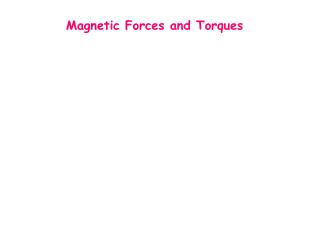DownloadDownload PresentationMagnetic Forces and Torques

Magnetic Forces and Torques

Télécharger la présentationMagnetic Forces and Torques

- - - - - - - - - - - - - - - - - - - - - - - - - - - E N D - - - - - - - - - - - - - - - - - - - - - - - - - - -
Presentation Transcript

1. Magnetic Forces and Torques

2. Review: Charged Particle in an external field: Straight wire of length L with current I in a uniform external field B: Note: L points in direction of positive current flow

3. Force on a current-carrying wire (general case) If B not uniform, and/or wire not straight: the force dF on a short segment of vector length dL is dF = IdL x B The total force on the wire is: dF B I Segment of length dL dL I

4. Example 1 • Find the force on: • The straight wire • The semicircular wire • The whole circuit y x x x x x x x x x I R x B (uniform) For (b): start with force dF due to an infinitesimal piece, and do the integral.

5. Solution

6. Theorem: For any closed current loop in a uniform magnetic field, Total magnetic force on the loop = 0 Proof: (if B is a constant vector) But: for a closed loop, so F = 0

7. Torque:Although there is no net force on a circuit in a uniform field, there may be a net torque. The torques due to equal and opposite forces applied at different locations do not necessarily cancel. We can calculate the torque directly for a rectangular loop. There is also a simple rule, which actually applies to a plane loop of any shape. First we need to define the “magnetic dipole moment” (a vector) for a current loop.

8. Magnetic Dipole Moment (or “magnetic moment”) Define the magnetic moment of a small current loop by current Area of loop Note the right-hand rule! – fingers follow I, thumb points in μ m

9. Torque on a Current Loop (Uniform B) Example: a rectangular loop B A h C D w Forces: FAB= I w B sinθ FAD= I h B FBC= I h B x FCD= I w B sinθ

10. Top view: FBC= I h B x B I into the page I w A w•sinθ FAD = I h B • Torque (about any pivot; e.g., at A)

11. The torque on a current loop with magnetic dipole momentin a uniform external field is: Where μ = IA

12. Example 2 Find the torque on a flat, horizontal, circular coil due to the magnetic field of the Earth. 50o north 0.5 x 10-4 T Circular loop, 2 turns, R = 1 m, I = 20 A, CCW (from above) Find: Torque (magnitude and direction)

13. Example 2 50o I Which direction does the torque vector point in for a flat horizontal coin on the surface of earth ?

14. Example 3 Find the torque on a flat, horizontal, circular coil due to the magnetic field of the Earth. 50o north 0.5 x 10-4 T Circular loop, 2 turns, R = 1 m, I = 20 A (ccw from above)

15. Example 4 Consider an electron orbiting a proton and maintained in a fixed circular path of radius R=5.29x10-11 m by the Coulomb force. Treating the orbiting charge as a current loop, calculate the resulting torque when the system is in an external magnetic field of B=0.4 T directed perpendicular to the magnetic moment of the orbiting electron.

16. Summary Charged Particle: Wire: Straight wire, Uniform B:Torque: